Anzeige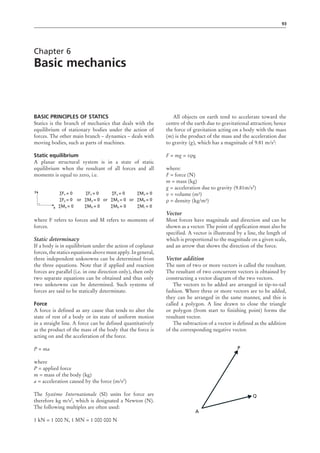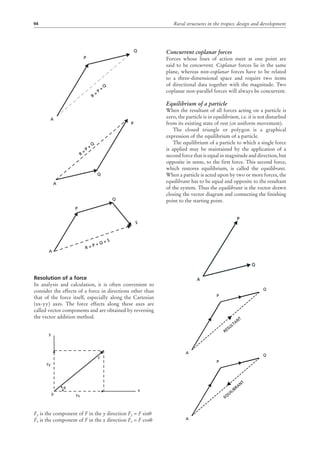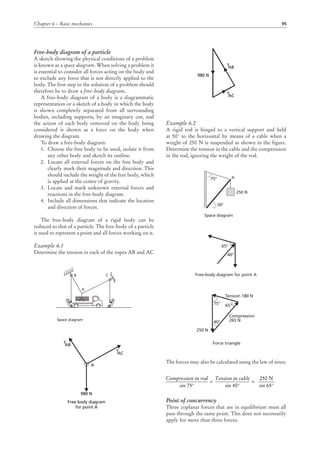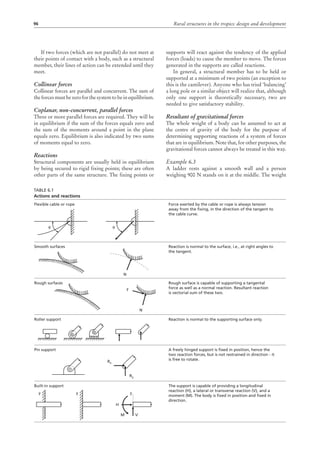Anzeige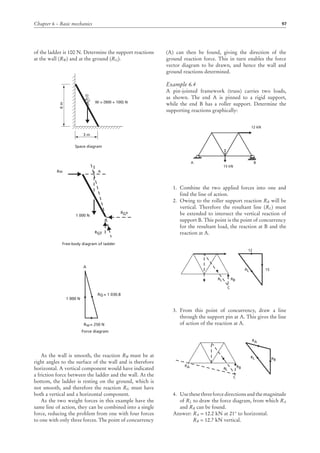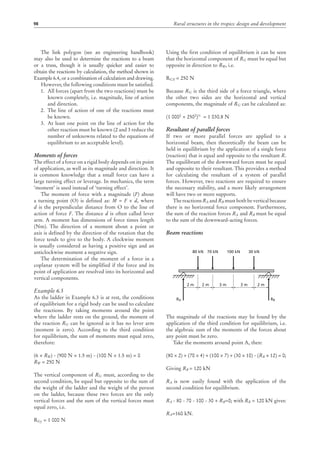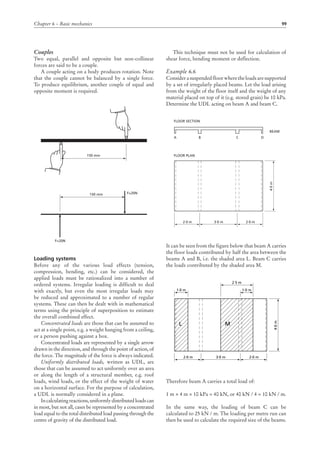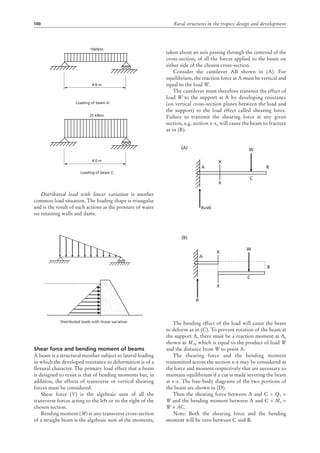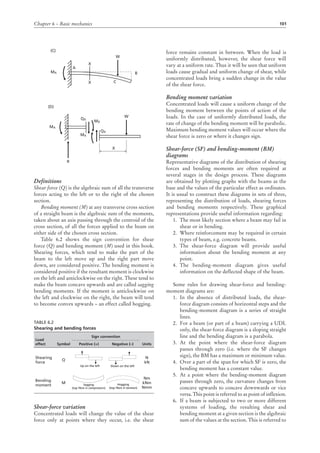Anzeige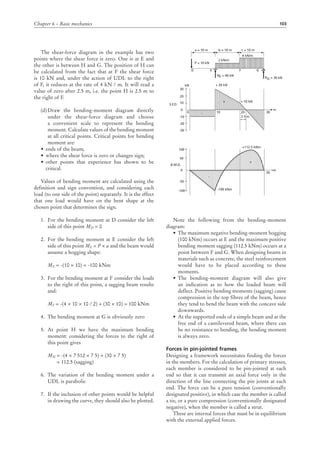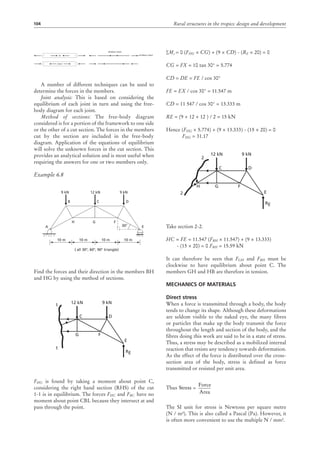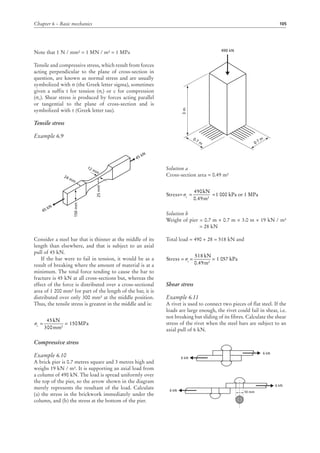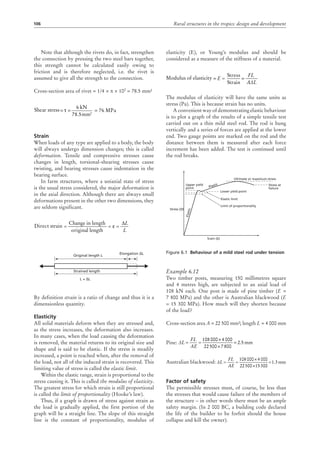Anzeige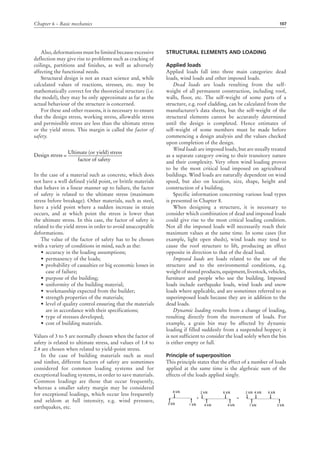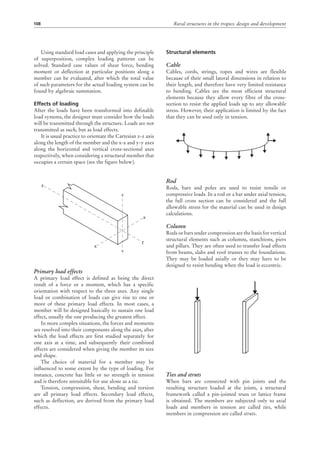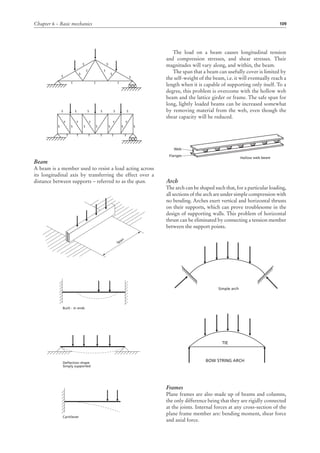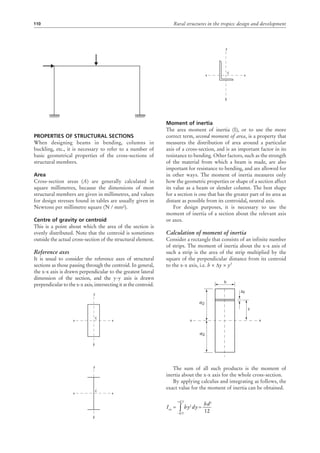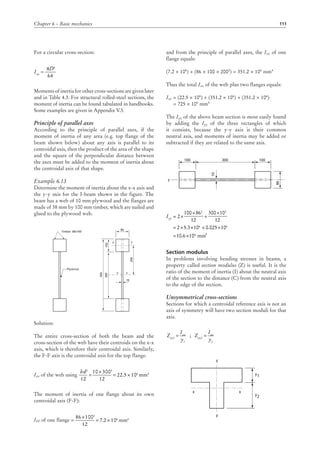Anzeige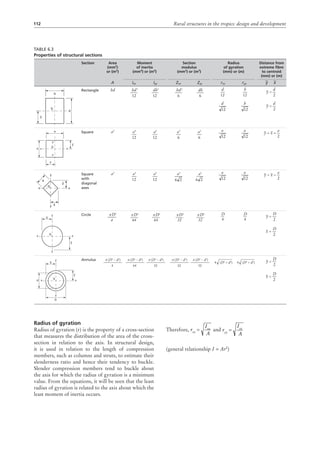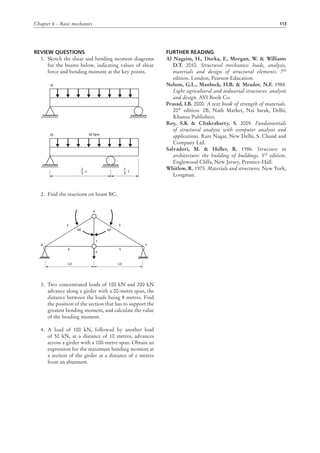Nächste SlideShare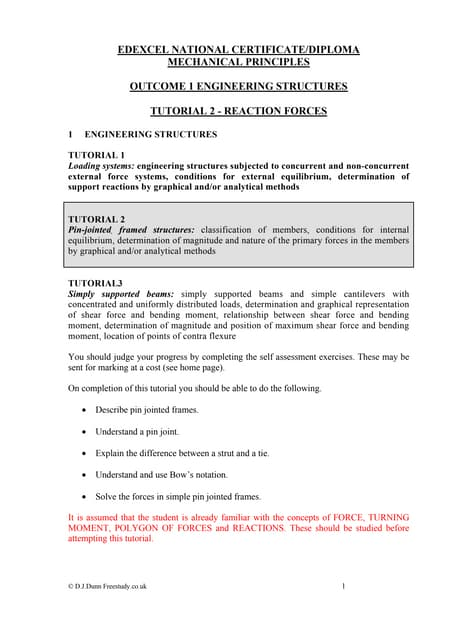Civil Engineering structure
1 von 22
Anzeige

### Momento en estructuras

1. 93 Chapter 6 Basic mechanics Basic principles of statics Statics is the branch of mechanics that deals with the equilibrium of stationary bodies under the action of forces. The other main branch – dynamics – deals with moving bodies, such as parts of machines. Static equilibrium A planar structural system is in a state of static equilibrium when the resultant of all forces and all moments is equal to zero, i.e. y x ∑Fx = 0 ∑Fy = 0 ∑Ma = 0 or ∑Fx = 0 ∑Ma = 0 ∑Mb = 0 or ∑Fy = 0 ∑Ma = 0 ∑Mb = 0 or ∑Ma = 0 ∑Mb = 0 ∑Mc = 0 where F refers to forces and M refers to moments of forces. Static determinacy If a body is in equilibrium under the action of coplanar forces, the statics equations above must apply. In general, three independent unknowns can be determined from the three equations. Note that if applied and reaction forces are parallel (i.e. in one direction only), then only two separate equations can be obtained and thus only two unknowns can be determined. Such systems of forces are said to be statically determinate. Force A force is defined as any cause that tends to alter the state of rest of a body or its state of uniform motion in a straight line. A force can be defined quantitatively as the product of the mass of the body that the force is acting on and the acceleration of the force. P = ma where P = applied force m = mass of the body (kg) a = acceleration caused by the force (m/s2 ) The Système Internationale (SI) units for force are therefore kg m/s2 , which is designated a Newton (N). The following multiples are often used: 1 kN = 1 000 N, 1 MN = 1 000 000 N All objects on earth tend to accelerate toward the centre of the earth due to gravitational attraction; hence the force of gravitation acting on a body with the mass (m) is the product of the mass and the acceleration due to gravity (g), which has a magnitude of 9.81 m/s2 : F = mg = vρg where: F = force (N) m = mass (kg) g = acceleration due to gravity (9.81m/s2 ) v = volume (m³) ρ = density (kg/m³) Vector Most forces have magnitude and direction and can be shown as a vector. The point of application must also be specified. A vector is illustrated by a line, the length of which is proportional to the magnitude on a given scale, and an arrow that shows the direction of the force. Vector addition The sum of two or more vectors is called the resultant. The resultant of two concurrent vectors is obtained by constructing a vector diagram of the two vectors. The vectors to be added are arranged in tip-to-tail fashion. Where three or more vectors are to be added, they can be arranged in the same manner, and this is called a polygon. A line drawn to close the triangle or polygon (from start to finishing point) forms the resultant vector. The subtraction of a vector is defined as the addition of the corresponding negative vector. A Q P
2. 94 Rural structures in the tropics: design and development Resolution of a force In analysis and calculation, it is often convenient to consider the effects of a force in directions other than that of the force itself, especially along the Cartesian (xx-yy) axes. The force effects along these axes are called vector components and are obtained by reversing the vector addition method. Fy is the component of F in the y direction Fy = F sinθ Fx is the component of F in the x direction Fx = F cosθ P Q A R = P + Q P Q A R = P + Q A P Q S R = P + Q + S y x Fy 0 F θ Fx Concurrent coplanar forces Forces whose lines of action meet at one point are said to be concurrent. Coplanar forces lie in the same plane, whereas non-coplanar forces have to be related to a three-dimensional space and require two items of directional data together with the magnitude. Two coplanar non-parallel forces will always be concurrent. Equilibrium of a particle When the resultant of all forces acting on a particle is zero, the particle is in equilibrium, i.e. it is not disturbed from its existing state of rest (or uniform movement). The closed triangle or polygon is a graphical expression of the equilibrium of a particle. The equilibrium of a particle to which a single force is applied may be maintained by the application of a second force that is equal in magnitude and direction, but opposite in sense, to the first force. This second force, which restores equilibrium, is called the equilibrant. When a particle is acted upon by two or more forces, the equilibrant has to be equal and opposite to the resultant of the system. Thus the equilibrant is the vector drawn closing the vector diagram and connecting the finishing point to the starting point. A Q P P Q A RESULTANT P Q A EQ UILIBRANT
3. 95Chapter 6 – Basic mechanics Free-body diagram of a particle A sketch showing the physical conditions of a problem is known as a space diagram. When solving a problem it is essential to consider all forces acting on the body and to exclude any force that is not directly applied to the body. The first step in the solution of a problem should therefore be to draw a free-body diagram. A free-body diagram of a body is a diagrammatic representation or a sketch of a body in which the body is shown completely separated from all surrounding bodies, including supports, by an imaginary cut, and the action of each body removed on the body being considered is shown as a force on the body when drawing the diagram. To draw a free-body diagram: 1. Choose the free body to be used, isolate it from any other body and sketch its outline. 2. Locate all external forces on the free body and clearly mark their magnitude and direction. This should include the weight of the free body, which is applied at the centre of gravity. 3. Locate and mark unknown external forces and reactions in the free-body diagram. 4. Include all dimensions that indicate the location and direction of forces. The free-body diagram of a rigid body can be reduced to that of a particle. The free-body of a particle is used to represent a point and all forces working on it. Example 6.1 Determine the tension in each of the ropes AB and AC B A Space diagram C ABT ABT ACT A 980 N Free body diagram for point A 980 N Example 6.2 A rigid rod is hinged to a vertical support and held at 50° to the horizontal by means of a cable when a weight of 250 N is suspended as shown in the figure. Determine the tension in the cable and the compression in the rod, ignoring the weight of the rod. The forces may also be calculated using the law of sines: Point of concurrency Three coplanar forces that are in equilibrium must all pass through the same point. This does not necessarily apply for more than three forces. ABT ACT A 980 N Free body diagram for point A 980 N 50° Space diagram 250 N 75° A 40° 65° Free-body diagram for point A 40° 65° Tension 180 N Compression 265 N 250 N 75° Force triangle sin 65° 250 N = sin 40° Tension in cable = sin 75° Compression in rod
4. 96 Rural structures in the tropics: design and development If two forces (which are not parallel) do not meet at their points of contact with a body, such as a structural member, their lines of action can be extended until they meet. Collinear forces Collinear forces are parallel and concurrent. The sum of the forces must be zero for the system to be in equilibrium. Coplanar, non-concurrent, parallel forces Three or more parallel forces are required. They will be in equilibrium if the sum of the forces equals zero and the sum of the moments around a point in the plane equals zero. Equilibrium is also indicated by two sums of moments equal to zero. Reactions Structural components are usually held in equilibrium by being secured to rigid fixing points; these are often other parts of the same structure. The fixing points or supports will react against the tendency of the applied forces (loads) to cause the member to move. The forces generated in the supports are called reactions. In general, a structural member has to be held or supported at a minimum of two points (an exception to this is the cantilever). Anyone who has tried ‘balancing’ a long pole or a similar object will realize that, although only one support is theoretically necessary, two are needed to give satisfactory stability. Resultant of gravitational forces The whole weight of a body can be assumed to act at the centre of gravity of the body for the purpose of determining supporting reactions of a system of forces that are in equilibrium. Note that, for other purposes, the gravitational forces cannot always be treated in this way. Example 6.3 A ladder rests against a smooth wall and a person weighing 900 N stands on it at the middle. The weight Table 6.1 Actions and reactions θ θ N N F Rx Ry y y y H M V Smooth surfaces Rough surfaces Roller support Pin support Built-in support Flexible cable or rope Reaction is normal to the surface, i.e., at right angles to the tangent. Rough surface is capable of supporting a tangental force as well as a normal reaction. Resultant reaction is vectorial sum of these two. Reaction is normal to the supporting surface only. A freely hinged support is ﬁxed in position, hence the two reaction forces, but is not restrained in direction - it is free to rotate. The support is capable of providing a longitudinal reaction (H), a lateral or transverse reaction (V), and a moment (M). The body is ﬁxed in position and ﬁxed in direction. Force exerted by the cable or rope is always tension away from the ﬁxing, in the direction of the tangent to the cable curve.
5. 97Chapter 6 – Basic mechanics of the ladder is 100 N. Determine the support reactions at the wall (RW) and at the ground (RG). As the wall is smooth, the reaction RW must be at right angles to the surface of the wall and is therefore horizontal. A vertical component would have indicated a friction force between the ladder and the wall. At the bottom, the ladder is resting on the ground, which is not smooth, and therefore the reaction RG must have both a vertical and a horizontal component. As the two weight forces in this example have the same line of action, they can be combined into a single force, reducing the problem from one with four forces to one with only three forces. The point of concurrency W = (900 + 100) N Space diagram 3 m 6m Rw A 1 000 N RGx RGy Free-body diagram of ladder A Rw= 250 N RG= 1 030.8 1 000 N Force diagram (A) can then be found, giving the direction of the ground reaction force. This in turn enables the force vector diagram to be drawn, and hence the wall and ground reactions determined. Example 6.4 A pin-jointed framework (truss) carries two loads, as shown. The end A is pinned to a rigid support, while the end B has a roller support. Determine the supporting reactions graphically: 1. Combine the two applied forces into one and find the line of action. 2. Owing to the roller support reaction RB will be vertical. Therefore the resultant line (RL) must be extended to intersect the vertical reaction of support B. This point is the point of concurrency for the resultant load, the reaction at B and the reaction at A. 3. From this point of concurrency, draw a line through the support pin at A. This gives the line of action of the reaction at A. 4. Usethesethreeforcedirectionsandthemagnitude of RL to draw the force diagram, from which RA and RB can be found. Answer: RA = 12.2 kN at 21° to horizontal. RB = 12.7 kN vertical. 12 kN 15 kN BA RL RL 15 12 RB C RL RL RB RB RA RA C
6. 98 Rural structures in the tropics: design and development The link polygon (see an engineering handbook) may also be used to determine the reactions to a beam or a truss, though it is usually quicker and easier to obtain the reactions by calculation, the method shown in Example 6.4, or a combination of calculation and drawing. However, the following conditions must be satisfied. 1. All forces (apart from the two reactions) must be known completely, i.e. magnitude, line of action and direction. 2. The line of action of one of the reactions must be known. 3. At least one point on the line of action for the other reaction must be known (2 and 3 reduce the number of unknowns related to the equations of equilibrium to an acceptable level). Moments of forces The effect of a force on a rigid body depends on its point of application, as well as its magnitude and direction. It is common knowledge that a small force can have a large turning effect or leverage. In mechanics, the term ‘moment’ is used instead of ‘turning effect’. The moment of force with a magnitude (F) about a turning point (O) is defined as: M = F × d, where d is the perpendicular distance from O to the line of action of force F. The distance d is often called lever arm. A moment has dimensions of force times length (Nm). The direction of a moment about a point or axis is defined by the direction of the rotation that the force tends to give to the body. A clockwise moment is usually considered as having a positive sign and an anticlockwise moment a negative sign. The determination of the moment of a force in a coplanar system will be simplified if the force and its point of application are resolved into its horizontal and vertical components. Example 6.5 As the ladder in Example 6.3 is at rest, the conditions of equilibrium for a rigid body can be used to calculate the reactions. By taking moments around the point where the ladder rests on the ground, the moment of the reaction RG can be ignored as it has no lever arm (moment is zero). According to the third condition for equilibrium, the sum of moments must equal zero, therefore: (6 × RW) - (900 N × 1.5 m) - (100 N × 1.5 m) = 0 RW = 250 N The vertical component of RG must, according to the second condition, be equal but opposite to the sum of the weight of the ladder and the weight of the person on the ladder, because these two forces are the only vertical forces and the sum of the vertical forces must equal zero, i.e. RGy = 1 000 N Using the first condition of equilibrium it can be seen that the horizontal component of RG must be equal but opposite in direction to RW, i.e. RGX = 250 N Because RG is the third side of a force triangle, where the other two sides are the horizontal and vertical components, the magnitude of RG can be calculated as: (1 0002 + 2502 )½ = 1 030.8 N Resultant of parallel forces If two or more parallel forces are applied to a horizontal beam, then theoretically the beam can be held in equilibrium by the application of a single force (reaction) that is equal and opposite to the resultant R. The equilibrant of the downward forces must be equal and opposite to their resultant. This provides a method for calculating the resultant of a system of parallel forces. However, two reactions are required to ensure the necessary stability, and a more likely arrangement will have two or more supports. The reactions RA and RB must both be vertical because there is no horizontal force component. Furthermore, the sum of the reaction forces RA and RB must be equal to the sum of the downward-acting forces. Beam reactions The magnitude of the reactions may be found by the application of the third condition for equilibrium, i.e. the algebraic sum of the moments of the forces about any point must be zero. Take the moments around point A, then: (80 × 2) + (70 × 4) + (100 × 7) + (30 × 10) - (RB × 12) = 0; Giving RB = 120 kN RA is now easily found with the application of the second condition for equilibrium. RA - 80 - 70 - 100 - 30 + RB=0; with RB = 120 kN gives: RA=160 kN. 80 kN 70 kN 100 kN 30 kN RA RB 2 m3 m3 m2 m2 m